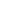SEARCH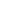BROWSEMorais, Joanna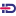, Thomas-Agnan, Christineand Simioni, Michel(2018) Interpreting of explanatory variables impacts in compositional regression models. Austrian Journal of Statistics, vol. 47 (n° 5). pp. 1-25.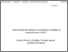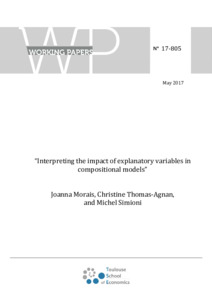Preview Text Download (1MB) | Preview
Identification Number : 10.17713/ajs.v47i5.718

## Abstract

Regression models have been developed for the case where the dependent variable is a vector of shares. Some of them, from the marketing literature, are easy to interpret but they are quite simple and can only be complexified at the expense of a very large number of parameters to estimate. Other models, from the mathematical literature, are called compositional regression models and are based on the simplicial geometry (a vector of shares is called a composition, shares are components, and a composition lies in the simplex). These models are transformation models: they use a log-ratio transformation of shares. They are very flexible in terms of explanatory variables and complexity (component-specific and cross-effect parameters), but their interpretation is not straightforward, due to the fact that shares add up to one. This paper combines both literatures in order to obtain a performing market-share model allowing to get relevant and appropriate interpretations, which can be used for decision making in practical cases. For example, we are interested in modeling the impact of media investments on automobile manufacturers sales. In order to take into account the competition, we model the brands market-shares as a function of (relative) media investments. We furthermore focus on compositional models where some explanatory variables are also compositional. Two specifications are possible: in Model A, a unique coefficient is associated to each compositional explanatory variable, whereas in Model B a compositional explanatory variable is associated to component-specific and cross-effect coefficients. Model A and Model B are estimated for our application in the B segment of the French automobile market, from 2003 to 2015. In order to enhance the interpretability of these models, we present different types of impact assessment measures (marginal effects, elasticities and odds ratios) and we show that elasticities are particularly useful to isolate the impact of an explanatory variable on a particular share. We show that elasticities can be equivalently computed from the transformed model and from the model in the simplex and that they are linked to directional C-derivatives of simplex-valued function of a simplex variable. Direct and cross effects of media investments are computed for both models. Model B shows interesting non-symmetric synergies between brands, and Renault seems to be the most elastic brand to its own media investments. In order to determine if component-specific and cross-effect parameters are needed to improve the quality of the model (Model B) or if a global parameter is reasonable (Model A), we compare the goodness-of-fit of the two models using (out-of-sample) quality measures adapted for share data.

Item Type: Article English 8 September 2018 Yes Elasticity, odds ratio, marginal effect, compositional model, compositional differential calculus, market-shares, media investments impact C10 - GeneralC25 - Discrete Regression and Qualitative Choice Models; Discrete RegressorsC35 - Discrete Regression and Qualitative Choice Models; Discrete RegressorsC46 - Specific Distributions; Specific StatisticsD12 - Consumer Economics - Empirical AnalysisM31 - Marketing B- ECONOMIE ET FINANCE TSE-R (Toulouse) UT1 10 Oct 2018 14:08 06 Sep 2021 13:02 oai:tse-fr.eu:32993 https://publications.ut-capitole.fr/id/eprint/26308